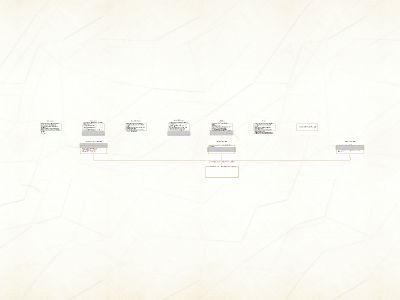Publications: 51 | Followers: 2

## Cryptography and Secret Codes - University of Arizona

Publish on Category: Birds 0

Cryptography and Secret Codes
or one reason that linear equations are cool.
Basic Cryptography Code
Romans would use this type of codeHere“LINEAR”becomes “OLQHDU”What would “HTXDWLRQ” be?
Numerical Code
We can do more if we change letters into numbers:So that “FWJH” becomes “6 23 10 8”Can you come up with another example?
Numerical Codes
Or we can even modify this code!Here “FWJH” becomes “9 26 13 11”Writing code like a table is hard though.Can you think of a simple rule to describe this code?
Linear Codes
The rule we came up with “Change it to a number and add 3” is a linear codeIt is more easily written with the linear equation y=x+3To encode “MUSTANGS” first change each letter to a number, and then apply the linear equationM1316;U2124; etc.
Linear codes
How would you write “MUSTANG ON” in the linear code y=2x+5?First turn it into numbers: “13 21 19 20 1 14 7 21 14”Then apply the linear code: “31 47 43 45 7 33 19 47 33”Now try with the linear code y=-3x+80How might we undo this?
Linear Block Codes
Linear block codes use more than one equation to make a code. How might you do this?First take a message “CRYPTOGRAPHY” and break it up into blocks.Here we will use block size 2, so CR-YP-TO-GR-AP-HY are the blocks.
Linear Block Codes
You then need as many linear equations as your block size.We need 2 equations, so let’s use y=2x+5 and y=-3x+80To make a code for CRYPTOGRAPHY, first take the first block ‘CR’ and encode it.C 3  2*3+5=11; R  18  -3*18+80=26So ‘CR’ becomes ‘11 26’. What is the rest of the code?
A Problem
Now encode “BOOT” using this block system.It becomes ‘9 35 35 20’The problem is that ‘O’ is encoded as ‘35’ for both rules. This is called collision.You can tell there is a double letter.To see this, solve the equation 2x+5=-3x+80 for x.
Review
Codes: Assign a new symbol to each letterNumerical Codes: Use numbers as the symbolLinear Codes: Use linear equations to change the numbersLinear Block Codes: Use more than one linear equation at a timeCollision: When the same letter is changed to the same number in a linear block code
Now go make your own code!

0

Embed

Share

Upload

Make amazing presentation for free
Cryptography and Secret Codes - University of Arizona